Technical Article

# Assessing Conductor and Dielectric Losses in Transmission Lines

September 15, 2023 by Dr. Steve Arar

## Understanding the causes of attenuation in a transmission line can help us choose the right solutions for our application. In this article, we’ll learn about attenuation caused by conductor loss and dielectric loss.

Electromagnetic waves are attenuated as they travel down a transmission line. In some cases, we may ignore this—if the losses are too small to matter, for example, or to simplify our analysis when establishing a transmission line’s baseline behavior. Nevertheless, the ability to assess a line’s attenuation can help us choose the right maximum trace length or the right low-loss material for our application.

The preceding article in this series introduced us to dielectric loss in transmission lines. This time, we’ll take a more in-depth look at how conductor and dielectric losses affect signal propagation along a transmission line.

### Loss Mechanisms in Transmission Lines

There are several different loss mechanisms that affect interconnects at high frequencies. These include:

• Mismatch loss. This occurs when the signal hits a discontinuity and a portion of the power reflects back toward the generator.
• Radiation loss. As the name suggests, this is energy lost due to RF radiation.
• Conductor loss and dielectric loss. Unlike the other loss types listed here, which only reflect or guide energy away from the transmission line, both conductor and dielectric loss absorb electromagnetic energy and convert it to heat.

### Lossy Components in the Transmission Line’s Model

Previously in this series, we learned about the lossy components of the transmission line model in Figure 1, namely the series resistance (R)  and the parallel conductance (G).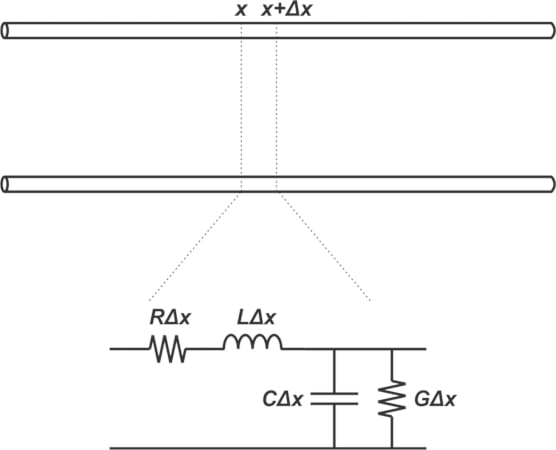##### Figure 1. Equivalent circuit of a two-conductor transmission line. Image used courtesy of Steve Arar

The skin effect increases the AC resistance in proportion to the square root of frequency. The conductance accounts for the dielectric loss, as described by Equation 1:

$G= C tan(\delta ) \omega$

##### Equation 1.

If we assume that the loss tangent, δ, is constant, the above equation shows that the conductance per unit length increases linearly with frequency (ω = 2πf).

### Equations of a Lossy Line

In a previous article, we derived the voltage and current equations for a lossless line. We can obtain the equations of a lossy line by following a similar procedure. We won’t go through the details of the derivation here; instead, let’s look at the final results. For a lossy line, the complex characteristic impedance (Z0) and the complex propagation constant (γ) are shown in Equations 2 and 3 below:

$Z_0 =\sqrt{\frac{R+jL \omega}{G+jC \omega}}$

##### Equation 2.

$\gamma = \alpha + j \beta = \sqrt{(R+jL \omega)(G+jC \omega)}$

##### Equation 3.

The propagation constant consists of two parts:

• The attenuation constant, ⍺, is the real part of γ and is measured in nepers per meter.
• The phase constant, β, is the unreal part of γ and is measured in radians per meter. It describes the phase shift per unit length.

If the line is along the x-axis, the forward-traveling voltage wave can take on the form:

$v(x,t)= A e^{-\alpha x} cos(\omega t-\beta x)$

##### Equation 4.

where A is a constant that can be found from boundary conditions at the input and output ports of the line. The major difference between the lossy and lossless cases is the exponential term e-⍺x, which shows that the signal gets attenuated as it travels along the lossy line.

### Simplifying ⍺ for a Low-Loss Line

A practical transmission line should have minimal losses; otherwise it would be useless for transmission of signals.We can see this by using Equation 1 to gauge the value of G. For a typical FR-4 board, the loss tangent is about 0.02, which leads to:

$G= 0.02 \times C \omega$

##### Equation 5.

Even for a lossy material, the conductance is only 1/50 of the admittance from the per-unit-length capacitance. Therefore, we can assume that:

$R \ll L \omega \quad and \quad G \ll C \omega$

##### Equation 6.

The above small-loss assumptions allow us to simplify different equations of the line. The characteristic impedance (Z0) can be approximated as:

$Z_0 \approx \sqrt{\frac{L}{C}}$

##### Equation 7.

Also, the propagation constant γ can be rewritten as:

$\begin{eqnarray} \gamma = \alpha + j \beta &=& \sqrt{(R+jL \omega)(G+jC \omega)} \\ &=&j \omega \sqrt{LC} \sqrt{(1+\frac{R}{jL\omega})(1+\frac{G}{jC\omega})} \end{eqnarray}$

##### Equation 8.

Finally, using the approximation $$\sqrt{1~+~x}~\simeq~1~+~\frac{x}{2}$$ for x ≪ 1, we can show that:

$\gamma = \alpha + j \beta \approx \frac{1}{2}(\frac{R}{Z_0}+GZ_0) + j \omega \sqrt{LC}$

##### Equation 9.

This is an important result that helps us understand how R and G in the line’s model contribute to the attenuation constant. For more information on this topic, I recommend the book “Transmission Lines in Digital and Analog Electric Systems: Signal Integrity and Crosstalk” by Clayton R. Paul.

### Decibels and Nepers

The ratio of two voltages can be expressed in two ways:

• Decibels. The most common way to express the ratio of two voltages, decibels use base 10, and are calculated using log10(V1/V2).
• Nepers. More rarely, voltage ratio is expressed in nepers, which use base e, the natural logarithm. Nepers are calculated using ln(V1/V2).

For example, an attenuation of 1 neper corresponds to a voltage ratio of e-1 = 0.37; in other words, a 1 neper attenuation means that the voltage at the end of the line is 0.37 times the initial voltage.

The following equation shows how the attenuation factor, α, expressed in nepers per meter, can be converted to dB per meter:

$\begin{eqnarray} Attenuation \ in \ dB &=& 20 log(e^{- \alpha}) \\ &=& -8.69 \times \alpha \end{eqnarray}$

##### Equation 10.

Note that the minus sign only shows that we have a loss rather than gain. The equation shows that a quantity in nepers can be expressed in decibels if we multiply it by 8.69; put a different way, 1 neper = 8.69 dB. When we apply the above conversion factor to Equation 9, the attenuation constant in dB per unit length is obtained as:

$\alpha \ [ dB \ per \ unit \ length] = 4.34(\frac{R}{Z_0}+GZ_0)$

##### Equation 11.

The first term on the right side of the above equation specifies the conductor loss in dB per unit length:

$\alpha_c = 4.34 \times \frac{R}{Z_0}$

##### Equation 12.

and the second term represents the dielectric loss (again in dB per unit length):

$\alpha_d = 4.34 \times G Z_0$

### Dielectric Loss and Conductor Dimensions

The characteristic impedance of a transmission line is related to the per-unit-length capacitance by the following equation:

$Z_0 = \frac{\sqrt{\epsilon_r}}{c \times C}$

##### Equation 14.

where c is the speed of light in a vacuum. Combining the above equation with the dielectric loss, ⍺d, yields:

$\alpha_d \ [ dB \ per \ inch] = 2.32 f \ tan(\delta) \sqrt{\epsilon_r}$

##### Equation 15.

where f is frequency in GHz. Note that the above equation gives dielectric loss in dB per inch.

Equation 15 hints at an interesting point: the dielectric loss is independent of capacitance (C). This is because G is directly proportional to C and Z0 is inversely proportional to C. Therefore, the dielectric loss—which is proportional to GZ0—is independent of C

This also means that the dielectric loss is independent of the line’s geometrical features, such as the PCB trace width in the case of a microstrip line. But does this mean that the loss of a PCB trace doesn’t change with its dimensions? Figure 2 shows the loss vs. trace width curves for two different types of materials.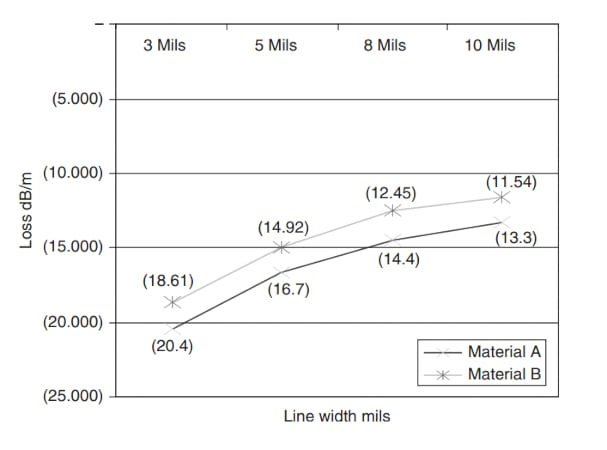##### Figure 2. Loss in relation to trace width for two types of materials. Image used courtesy of Clyde Coombs

According to Equation 15, ⍺d is independent of the conductor dimensions. However, increasing the trace width reduces the other important loss term: the conductor loss. As we discussed earlier in this series of articles, the AC resistance of a line with resistivity ρ can be approximated by:

$R_{AC}\approx \frac{\rho}{p \delta} \quad \Omega /m$

##### Equation 16.

In the above equation, p denotes the perimeter of the conductor and δ is the skin depth. By using a wider trace, p is increased, which consequently reduces the AC resistance (RAC) and the conductor loss (c). Note that the use of wide traces can negatively impact the circuit density.

### Loss Variation with Frequency

We know that the skin depth δ is inversely proportional to the square root of frequency:

$\delta = \frac{1}{\sqrt{\pi f \mu \sigma}}$

##### Equation 17.

Therefore, Equations 12 and 16 show that the conductor loss increases with the square root of frequency. On the other hand, the dielectric loss is directly proportional to frequency (Equation 15).

At low- and mid-band frequencies, the dielectric loss is very small and the overall loss is determined by the skin effect. At higher frequencies, however, the dielectric loss takes over because it grows faster than the conductor loss. Figure 3 shows the conductor loss and the dielectric loss, as well as the combined effect of these two loss terms for a 40-cm trace on a typical FR-4 substrate.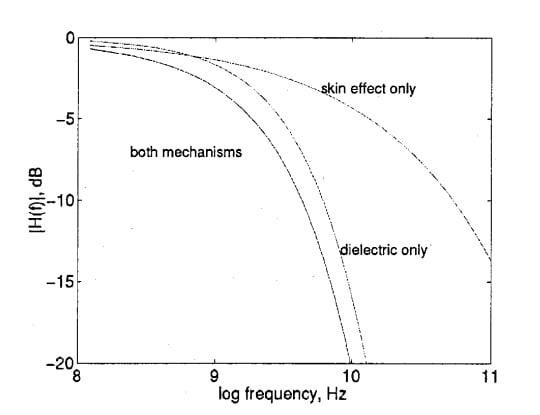##### Figure 3. Conductor loss, dielectric loss, and the combined effect of both for a 40-cm trace. Image used courtesy of C. Svensson

As we can see, the dielectric loss is smaller than the conductor loss at lower frequencies; at higher frequencies, however, it becomes the dominant mechanism. If we use logarithmic scales for both the attenuation and frequency axes, the curve should have a slope of about ½ when the skin effect is dominant and a slope of 1 when the dielectric effect takes over. You’ll commonly see the loss terms represented by straight lines, as they are in Figure 4.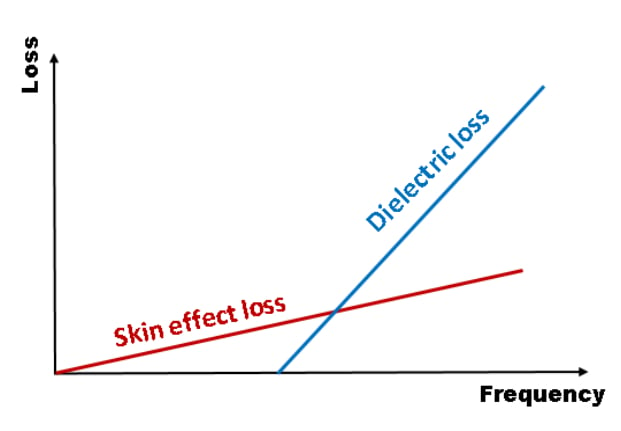##### Figure 4. Skin effect loss and dielectric loss. Image used courtesy of Y. Liao

As an example, Figure 5 illustrates the attenuation of a 50-Ω microstrip with an 8-mil-wide trace on a FR-4 board.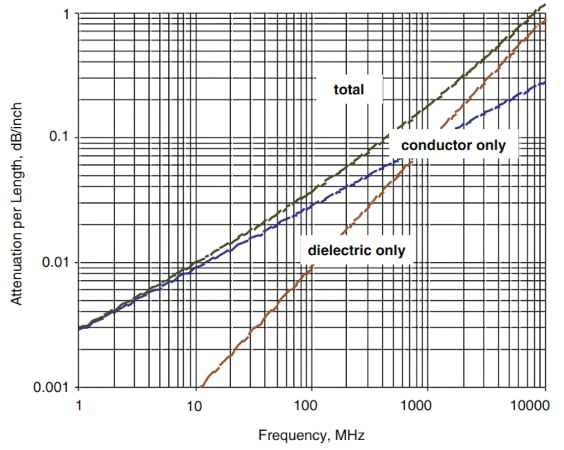### Other Performance Regions of a Transmission Line

Interconnect modeling has been well explored in the existing literature. In the above discussion, we learned about the skin effect and dielectric limited region of operation of transmission lines. Depending on the line’s length, the frequency of operation, and the value of different parameters in the RLGC model of the line, we can have lines that operate in a different way.

For example, integrated circuits use conductors with much smaller cross-sectional areas. This can make the inductive reactance of the line insignificant in comparison to the DC resistance of the line. In this case, the line operates in a region known as the RC region. In this region, the line’s response is totally different. The characteristic impedance in the RC region is given by:

$Z_c \approx \sqrt{\frac{R}{j \omega C}}$

##### Equation 18.

The characteristic impedance in the skin effect and dielectric limited regions (Equation 7) is a constant value, which makes providing a matched load straightforward. However, the characteristic impedance of the RC region is dependent on frequency. This means that it isn’t easy to provide a match in this mode of operation if we need to. Fortunately, a PCB trace isn’t likely to exhibit any observable RC mode phenomenon.

The lumped-element region and the LC region represent two other modes of operation. We won’t discuss them today, but I recommend Dr. Howard Johnson’s “High Speed Signal Propagation: Advanced Black Magic” if you want a detailed treatment of the topic. Additionally, the book “Circuits, Interconnections and Packaging for VLSI” by H. B. Bakoglu provides in-depth coverage of interconnect issues in integrated circuits.

Featured image used courtesy of Adobe Stock